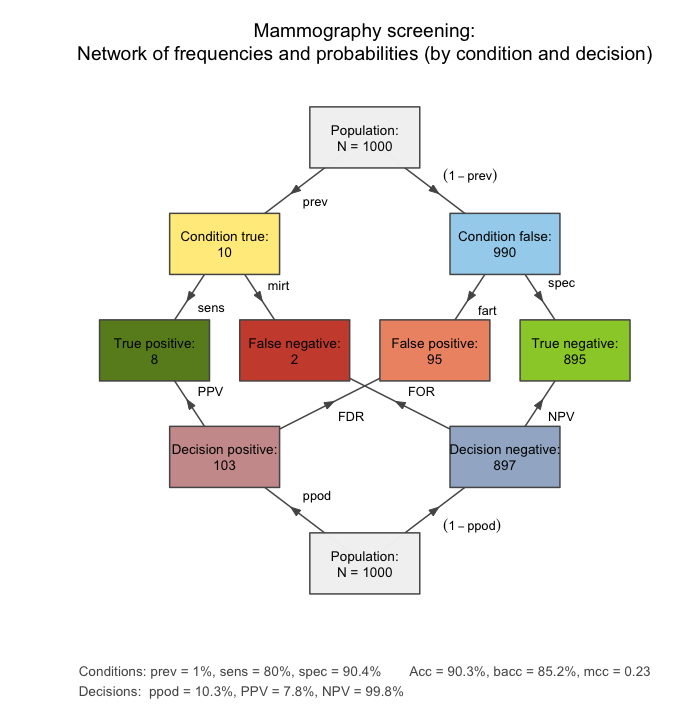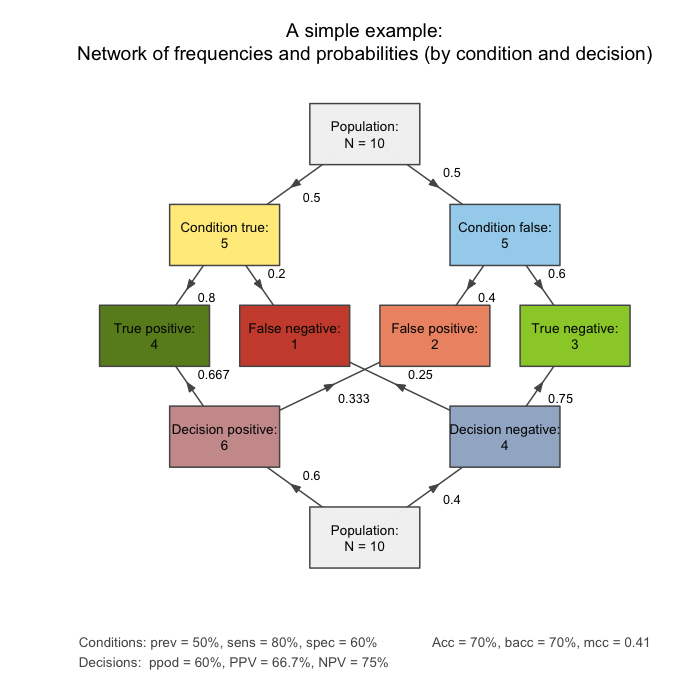# riskyr Data Formats

#### 2018 02 05

The quest for certainty is the biggest obstacle to becoming risk savvy. (Gerd Gigerenzer)1

A major challenge in mastering risk literacy is coping with inevitable uncertainty. Fortunately, uncertainty in the form of risk can be expressed in terms of probabilities and thus be measured and calculated — or “reckoned” — with (Gigerenzer, 2002). Nevertheless, probabilities are pretty big obstacles for everyone not trained as a statistician. As probabilities are notoriously difficult to communicate and understand, this vignette explains how probabilities can be defined and computed in terms of frequencies.

## Two Representational Formats

The problems addressed by riskyr and the scientific discussion surrounding them is framed in terms of two representational formats: frequencies are distinguished from probabilities. (See the user guide for background and references.)

riskyr reflects this division by distinguishing between the same two data types and hence provides objects that contain frequencies (specifically, a list called freq) and objects that contain probabilities (a list called prob). But before we explain their contents, it is important to realize that any such separation is an abstract and artificial one. It may make sense to distinguish frequencies from probabilities for conceptual and educational reasons, but both in theory and in reality both representations are intimately intertwined.

In the following, we will first consider frequencies and probabilities by themselves, but then show how both are related. As a sneak preview, the following network plot shows frequencies as its nodes and probabilities as the edges that link the nodes:

library("riskyr") # load the "riskyr" package

plot_fnet(prev = .01, sens = .80, spec = NA, fart = .096,  # 3 essential probabilities
N = 1000,       # 1 frequency
area = "no",    # all boxes have the same size
p.lbl = "nam",  # show probability names on edges
title.lbl = "Mammography screening")A network diagram that shows frequencies as nodes and probabilities as edges between nodes.

## Frequencies

For our purposes, frequencies simply are numbers that can be counted — either 0 or positive integers.2

### Definitions

The following 11 frequencies are distinguished by riskyr and contained in freq:

Nr. Variable Definition
1. N The number of cases (or individuals) in the population.
2. cond.true The number of cases for which the condition is present (TRUE).
3. cond.false The number of cases for which the condition is absent (FALSE).
4. dec.pos The number of cases for which the decision is positive (TRUE).
5. dec.neg The number of cases for which the decision is negative (FALSE).
6. dec.cor The number of cases for which the decision is correct (correspondence of decision to condition).
7. dec.err The number of cases for which the decision is erroneous (difference between decision and condition).
8. hi The number of hits or true positives: condition present (TRUE) & decision positive (TRUE).
9. mi The number of misses or false negatives: condition present (TRUE) & decision negative (FALSE).
10. fa The number of false alarms or false positives: condition absent (FALSE) & decision positive (TRUE).
11. cr The number of correct rejections or true negatives: condition absent (FALSE) & decision negative (FALSE).

### Perspectives: Basic vs. combined frequencies

The frequencies contained in freq can be viewed from two perspectives:

• From the entire population to different parts or subsets (top-down):
Whereas N specifies the size of the entire population, the other 10 frequencies denote the number of individuals or cases in some subset. For instance, the frequency dec.pos denotes individuals for which the decision or diagnosis is positive. As this frequency is contained within the population, its numeric value must range from 0 to N.

• From four essential subgroups to various combinations of them (bottom-up):
As the four frequencies hi, mi, fa, and cr are not further split into subgroups, we can think of them as atomic elements or four essential frequencies. All other frequencies in freq are sums of various combinations of these four essential frequencies. This implies that the entire network of frequencies and probabilities (shown in the network diagram above) can be reconstructed from these four essential frequencies.

### Relationships among frequencies

The following relationships hold among the 11 frequencies:

1. The population size N can be split into several subgroups by classifying individuals by four different criteria:

1. by condition
1. by decision
1. by the correspondence of decisions to conditions
1. by the actual combination of condition and decision

Depending on the criterion used, the following relationships hold:

\begin{aligned} \texttt{N} &= \texttt{cond.true} + \texttt{cond.false} & \textrm{(a)}\\ &= \texttt{dec.pos} + \texttt{dec.neg} & \textrm{(b)}\\ &= \texttt{dec.cor} + \texttt{dec.err} & \textrm{(c)}\\ &= \texttt{hi} + \texttt{mi} + \texttt{fa} + \texttt{cr} & \textrm{(d)}\\ \end{aligned}

Similarly, each of the subsets resulting from using the splits by condition, by decision, or by their correspondence, can also be expressed as a sum of two of the four essential frequencies. This results in three different ways of grouping the four essential frequencies:

1. by condition (corresponding to the two columns of the confusion matrix):

\begin{aligned} \texttt{N} \ &= \ \texttt{cond.true} & +\ \ \ \ \ &\texttt{cond.false} & \textrm{(a)} \\ \ &= \ (\texttt{hi} + \texttt{mi}) & +\ \ \ \ \ &(\texttt{fa} + \texttt{cr}) \\ \end{aligned}

1. by decision (corresponding to the two rows of the confusion matrix):

\begin{aligned} \texttt{N} \ &= \ \texttt{dec.pos} & +\ \ \ \ \ &\texttt{dec.neg} & \ \ \ \ \ \textrm{(b)} \\ \ &= \ (\texttt{hi} + \texttt{fa}) & +\ \ \ \ \ &(\texttt{mi} + \texttt{cr}) \\ \end{aligned}

1. by the correspondence of decisions to conditions (corresponding to the two diagonals of the confusion matrix):

\begin{aligned} \texttt{N} \ &= \ \texttt{dec.cor} & +\ \ \ \ \ &\texttt{dec.err} & \ \ \ \ \textrm{(c)} \\ \ &= \ (\texttt{hi} + \texttt{cr}) & +\ \ \ \ \ &(\texttt{mi} + \texttt{fa}) \\ \end{aligned}

It may be tempting to refer to instances of dec.cor and dec.err as “true decisions” and “false decisions”. However, this would invite conceptual confusion, as “true decisions” actually include cond.false cases (cr) and “false decisions” actually include cond.true cases (mi).

## Probabilities

The notions of probability is as elusive as ubiquitous (see Hájek, 2012, for a solid exposition of its different concepts and interpretations). For our present purposes, probabilities are simply numbers between 0 and 1. These numbers are defined to reflect particular quantities and can be expressed as percentages or fractions of other numbers (frequencies or probabilities).

### Definitions

The following 10 probabilities are distinguished by riskyr and contained in prob:

Nr. Variable Name Definition
1. prev prevalence The probability of the condition being TRUE.
2. sens sensitivity The conditional probability of a positive decision provided that the condition is TRUE.
3. mirt miss rate The conditional probability of a negative decision provided that the condition is TRUE.
4. spec specificity The conditional probability of a negative decision provided that the condition is FALSE.
5. fart false alarm rate The conditional probability of a positive decision provided that the condition is FALSE.
6. ppod proportion of positive decisions The proportion (baseline probability or rate) of the decision being positive (but not necessarily TRUE).
7. PPV positive predictive value The conditional probability of the condition being TRUE provided that the decision is positive.
8. FDR false detection rate The conditional probability of the condition being FALSE provided that the decision is positive.
9. NPV negative predictive value The conditional probability of the condition being FALSE provided that the decision is negative.
10. FOR false omission rate The conditional probability of the condition being TRUE provided that the decision is negative.

### Non-conditional vs. conditional probabilities

Note that there are only two non-conditional probabilities:

• The prevalence prev (1.) only depends on features of the condition.
• The proportion of positive decisions ppod (6.) only depends on features of the decision.

The other eight probabilities are conditional probabilities:

• Four conditional probabilities (2. to 5.) depend on the condition’s prev and features of the decision.
• Four conditional probabilities (7. to 10.) depend on the decision’s ppod and features of the condition.

### Relationships among probabilities

The following relationships hold among the conditional probabilities:

• The sensitivity sens and miss rate mirt are complements:

$\texttt{sens} = 1 - \texttt{mirt}$ - The specificity spec and false alarm rate fart are complements:

$\texttt{spec} = 1 - \texttt{fart}$ - The positive predictive value PPV and false detection rate FDR are complements:

$\texttt{PPV} = 1 - \texttt{FDR}$ - The negative predictive value NPV and false omission rate FOR are complements:

$\texttt{NPV} = 1 - \texttt{FOR}$

It is possible to adapt Bayes’ formula to define PPV and NPV in terms of prev, sens, and spec:

$\texttt{PPV} = \frac{\texttt{prev} \cdot \texttt{sens}}{\texttt{prev} \cdot \texttt{sens} + (1 - \texttt{prev}) \cdot (1 - \texttt{sens})}\\ \\ \\ \texttt{NPV} = \frac{(1 - \texttt{prev}) \cdot \texttt{spec}}{\texttt{prev} \cdot (1 - \texttt{sens}) + (1 - \texttt{prev}) \cdot \texttt{spec}}$

Although this is how the functions comp_PPV and comp_NPV compute the desired conditional probability, it is difficult to remember and think in these terms. Instead, we recommend thinking about and defining all conditional probabilities in terms of frequencies (see below).

## Probabilities as Ratios of Frequencies

The easiest way to think about, define, and compute the probabilities (contained in prob) are in terms of frequencies (contained in freq):

Nr. Variable Name Definition as Frequencies
1. prev prevalence The probability of the condition being TRUE. prev = cond.true/N
2. sens sensitivity The conditional probability of a positive decision provided that the condition is TRUE. sens = hi/cond.true
3. mirt miss rate The conditional probability of a negative decision provided that the condition is TRUE. mirt = mi/cond.true
4. spec specificity The conditional probability of a negative decision provided that the condition is FALSE. spec = cr/cond.false
5. fart false alarm rate The conditional probability of a positive decision provided that the condition is FALSE. fart = fa/cond.false
6. ppod proportion of positive decisions The proportion (baseline probability or rate) of the decision being positive (but not necessarily TRUE). ppod = dec.pos/N
7. PPV positive predictive value The conditional probability of the condition being TRUE provided that the decision is positive. PPV = hi/dec.pos
8. FDR false detection rate The conditional probability of the condition being FALSE provided that the decision is positive. FDR = fa/dec.pos
9. NPV negative predictive value The conditional probability of the condition being FALSE provided that the decision is negative. NPV = cr/dec.neg
10. FOR false omission rate The conditional probability of the condition being TRUE provided that the decision is negative. FOR = mi/dec.neg

Note that the ratios of frequencies are straightforward consequences of the probabilities’ definitions:

1. The two unconditional probabilities (1. and 6.) are proportions of the entire population:
• prev = cond.true/N
• ppod = dec.pos/N
1. All conditional probabilities (2.–5. and 7.–10.) can be computed as a proportion of the reference group on which they are conditional. More specifically, if we schematically read each definition as “The conditional probability of $$X$$ provided that $$Y$$”, then the ratio of the corresponding frequencies is X & Y/Y. More explicitly,
• the ratio’s numerator is the frequency of the joint occurrence (i.e., both X & Y) being the case;
• the ratio’s denominator is the frequency of the condition (Y) being the case.

## Exercise

### An example

The following network diagram is based on the following inputs:

• a condition’s prevalence of 50% (prev = .50);
• a decision’s sensitivity of 80% (sens = .80);
• a decision’s specificity of 60% (spec = .60);
• a population size of 10 individuals (N = 10);

and illustrates the relationship between frequencies and probabilities:

plot_fnet(prev = .50, sens = .80, spec = .60,  # 3 essential probabilities
N = 10,         # 1 frequency
area = "no",    # all boxes have the same size
p.lbl = "num",  # show numeric probability values on edges
title.lbl = "A simple example")An example network diagram that shows how probabilities can be computed as ratios of frequencies.

2. What is the frequency of dec.cor and dec.err cases? Where do these cases appear in the diagram?